# NCERT Solutions for Chapter 11 Algebra Class 6 Maths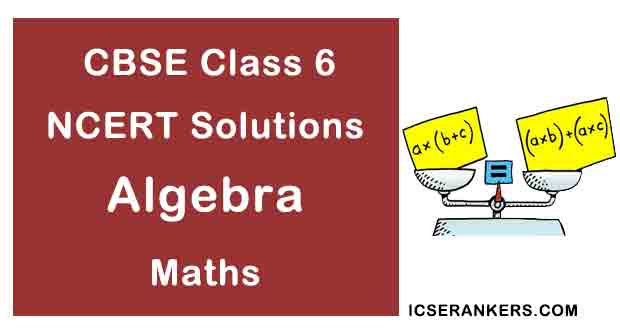Chapter Name Algebra NCERT Solutions Class CBSE Class 6 Textbook Name Maths Related Readings NCERT Solutions for Class 6NCERT Solutions for Class 6 Maths Summary of Algebra

Exercise 11.1

Question 1: Find the rule, which gives the number of matchsticks required to make the following matchsticks patterns. Use a variable to write the rule.

(a) A pattern of letter T as(b) A pattern of letter Z as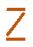(c) A pattern of letter U as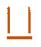(d) A pattern of letter V as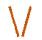(e) A pattern of letter E as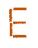(f) A pattern of letter S as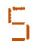(g) A pattern of letter A as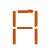Solution

(a) Pattern of letter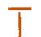= 2n (as two matchstick used in each letter)

(b) Pattern of letter= 3n (as three matchstick used in each letter)

(c) Pattern of letter= 3n (as three matchstick used in each letter)

(d) Pattern of letter= 2n (as two matchstick used in each letter)

(e) Pattern of letter= 5n (as five matchstick used in each letter)

(f) Pattern of letter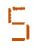= 5n (as five matchstick used in each letter)

(g) Pattern of letter= 6n (as six matchstick used in each letter)

Question 2: We already know the rule for the pattern of letter L, C and F. Some of the letters from Q.1 (given above) give us the same rule as that given by L. Which are these? Why does this happen?

Solution

The letter ‘T’ and ‘V’ that has pattern 2n, since 2 matchsticks are used in all these letters.

Question 3: Cadets are marching in a parade. There are 5 cadets in a row. What is the rule, which gives the number of cadets, given the number of rows? (Use n for the number of rows)

Solution

Number of rows = n

Cadets in each row = 5

Therefore, total number of cadets = 5n

Question 4: If there are 50 mangoes in a box, how will you write the total number of mangoes in terms of the number of boxes? (Use b for the number of boxes)

Solution

Number of boxes = b

Number of mangoes in each box = 50

Therefore, total number of mangoes = 50b

Question 5: The teacher distributes 5 pencils per student. Can you tell how many pencils are needed, given the number of students? (Use s for the number of students)

Solution

Number of students = s

Number of pencils to each student = 5

Therefore, total number of pencils needed are = 5s

Question 6: A bird flies 1 kilometer in one minute. Can you express the distance covered by the bird in terms of its flying time in minutes? (Use t for flying time in minutes)

Solution

Time taken by bird = t minutes

Speed of bird = 1 km per minute

Therefore, Distance covered by bird = speed × time = 1 × t = t km

Question 7: Radha is drawing a dot Rangoli (a beautiful pattern of lines joining dots with chalk powder as in figure). She has 8 dots in a row. How many dots will her Rangoli have for r rows? How many dots are there if there are 8 rows? If there are 10 rows?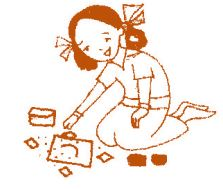Solution

Number of dots in each row = 8 dots

Number of rows = r

Therefore, number of dots = 8r

When there are 8 rows, then number of dots = 8 × 8 = 64 dots

When there are 10 rows, then number of dots = 8 × 10 = 80 dots

Question 8: Leela is Radha’s younger sister. Leela is 4 years younger than Radha. Can you write Leela’s age in terms of Radha’s age? Take Radha’s age to be x years.

Solution

Therefore, Leela’s age = (x – 4) years

Question 9: Mother has made laddus. She gives some laddus to guests and family members; still 5 laddus remain. If the number of laddus mother gave away is l, how many laddus did she make?

Solution

Number of laddus gave away = l

Number of laddus remaining = 5

Total number of laddus = (l + 5)

Question 10: Oranges are to be transferred from larger boxes into smaller boxes. When a large box is emptied, the oranges from it fill two smaller boxes and still 10 oranges remain outside. If the number of oranges in a small box are taken to be x, what is the number of oranges in the larger box?

Solution

Number of oranges in one box = x

Number of boxes = 2

Therefore, total number of oranges in boxes = 2x

Remaining oranges = 10

Thus, number of oranges = 2x + 10

Question 11: (a) Look at the following matchstick pattern of squares. The squares are not separate. Two neighbouring squares have a common matchstick. Observe the patterns and find the rule that gives the number of matchsticks in terms of the number of squares. (Hint: If you remove the vertical stick at the end, you will get a pattern of Cs.)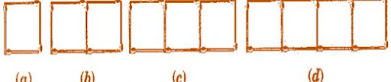(b) Figs. Below gives a matchstick pattern of triangles. As in Exercise 11 (a) above find the general rule that gives the number of matchsticks in terms of the number of triangles.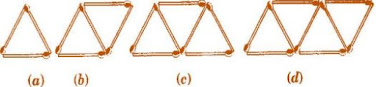Solution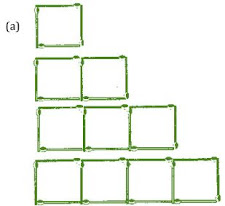If we remove 1 from each then they makes table of 3, i.e., 3, 6, 9, 12... So the required equation = 3x + 1 , where x is number of squares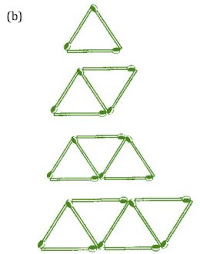If we remove 1 from each then they makes table of 2, i.e., 2, 4, 6, 8... So the required equation = 2x + 1 , where x is number of triangles..

Exercise 11.2

Question 1: The side of an equilateral triangle is shown by l. Express the perimeter of the equilateral triangle using l.

Solution

Side of equilateral triangle = l

Therefore, Perimeter of equilateral triangle = 3 × side = 3l

Question 2: The side of a regular hexagon is denoted by l. Express the perimeter of the hexagon using l. (Hint: A regular hexagon has all its six sides in length)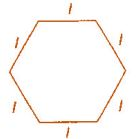Solution

Side of hexagon = l

Therefore, Perimeter of Hexagon = 6 × side = 6l

Question 3: A cube is a three-dimensional figure. It has six faces and all of them are identical squares. The length of an edge of the cube is given by l. find the formula for the total length of the edges of a cube.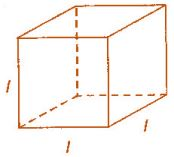Solution

Length of one edge of cube = l

Number of edges in a cube = 12

Therefore, total length = 12 × l = 12l

Question 4: The diameter of a circle is a line, which joins two points on the circle and also passes through the centre of the circle. (In the adjoining figure AB is a diameter of the circle; C is its centre). Express the diameter of the circle (d) in terms of its radius (r).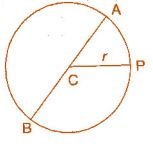Solution

Since, length of diameter is double the length of radius.

Therefore, d = 2r

Question 5: To find sum of three numbers 14, 27 and 13. We can have two ways.

(a) We may first add 14 and 27 to get 41 and then add 13 to it to get the total sum 54, or

(b) We may add 27 and 13 to get 40 and then add 14 to get the sum 54. Thus

(14 + 27) + 13 = 14 + (27 + 13)

This can be done for any three numbers. This property is known as the associativity of addition of numbers. Express this property which we have already studied in the chapter on Whole Numbers, in a general way, by using variables a, b and c.

Solution

(a + b) + c = a + (b + c)

Exercise 11.3

Question 1: Make up as many expressions with numbers (no variables) as you can from three numbers 5, 7 and 8. Every number should be used not more than once. Use only addition, subtraction and multiplication. (Hint: Three possible expressions are 5 + (8 – 7), 5 – (8 – 7), (5 x 8) + 7 make the other expressions)

Solution

(a) (8 × 5) – 7

(b) (8 + 5) – 7

(c) (8 × 7) – 5

(d) (8 + 7) – 5

(e) 5 × (7 + 8)

(f) 5 + (7 x 8)

(g) 5 + (8 – 7)

(h) 5 – (7 + 8)

Question 2: Which out of the following are expressions with numbers only:

(a) y + 3

(b) (7 × 20) – 8z

(c) 5 (21 – 7) + 7 × 2

(d) 5

(e) 3x

(f) 5 – 5n

(g) (7 × 20) – (5 × 10) – 45 + p

Solution

(c) and (d)

Question 3: Identify the operations (addition, subtraction, division, multiplication) in forming the following expressions and tell how the expressions have been formed:

(a) z + 1, z – 1, y + 17, y – 17

(b) 17y, y/17, 5z

(c) 2y + 17, 2y – 17

(d) 7m, - 7m + 3, - 7m – 3

Solution

z – 1→ Subtraction

y – 17→ Subtraction

(b) 17y→ Multiplication

y/17→ Division

5z→ Multiplication

(c) 2y + 17→ Multiplication and Addition

2y – 17→ Multiplication and Subtraction

(d) 7m→ Multiplication

- 7m + 3→ Multiplication and Addition

- 7m – 3→ Multiplication and Subtraction

Question 4: Give expressions for the following cases:

(b) 7 subtracted from p.

(c) p multiplied by 7.

(d) p divided by 7.

(e) 7 subtracted from -m.

(f)  -p multiplied by 5.

(g)  -p divided by 5.

(h) p multiplied by -5.

Solution

(a) p +7

(b) p – 7

(c) 7p

(d) p/7

(e)  -m-7

(f) -5p

(g) –p/5

(h) -5p

Question 5: Give expression in the following cases:

(b) 11 subtracted from 2m

(c) 5 times y to which 3 is added.

(d) 5 times y from which 3 is subtracted.

(e) y is multiplied by -8.

(f) y is multiplied by -8 and then 5 is added to the result.

(g) y is multiplied by 5 and result is subtracted from 16.

(h) y is multiplied by -5 and the result is added to 16.

Solution

(a) 2m + 11

(b) 2m – 11

(b) 5y + 3

(d) 5y – 3

(e) – 8y

(f) – 8y + 5

(g) 16 – 5y

(h) – 5y + 16

Question 6: (a) From expressions using t and 4. Use not more than one number operation. Every expression must have t in it.

(b) Form expressions using y, 2 and 7. Every expression must have y in it. Use only two number operations. These should be different.

Solution

(a) t + 4, t – 4, 4 – t, 4t, t/4, 4/t

(b) 2y + 7, 2y – 7, 7y = 2, 7y – 2 and so on.

Exercise 11.4

(a) Take Sarita’s present age to be y years.

(i) What will be her age 5 years from now?

(ii) What was her age 3 years back?

(iii) Sarita’s grandfather is 6 times her age. What is the age of her grandfather?

(iv) Grandmother is 2 years younger than grandfather. What is grandmother’s age?

(v) Sarita’s father’s age is 5 years more than 3 times Sarita’s age. What is her father’s age?

(b) The length of a rectangular hall is 4 meters less than 3 times the breadth of the hall. What is the length, if the breadth is b meters?

(c) A rectangular box has height h cm. Its length is 5 times the height and breadth is 10 cm less than the length. Express the length and the breadth of the box in terms of the height.

(d) Meena, Beena and Leena are climbing the steps to the hill top. Meena is at step s, Beena is 8 steps ahead and Leena 7 steps behind. Where are Beena and Meena? The total number of steps to the hill top is 10 less than 4 times what Meena has reached. Express the total number of steps using s.

(e) A bus travels at v km per hour. It is going from Daspur to Beespur. After the bus has travelled 5 hours. Beespur is still 20 km away. What id the distance from Daspur to Beespur? Express it using v.

Solution

(a) (i) y + 5

(ii) y - 3

(iii) 6y

(iv) 6y - 2

(v) 3y + 5

(b) Length = 3b and Breadth = (3b – 4) meters

(c) Height of the box = h cm

Length of the box = 5 times the height = 5h cm

Breadth of the box = 10 cm less than length = (5h – 10) cm

(d) Meena’s position = s

Beena’s position = 8 steps ahead = s + 8

Leena’s position = 7 steps behind = s - 7

Total number of steps = 4s - 10

(e) Speed of the bus = v km/h

Distance travelled in 5 hours = 5v km

Remaining distance = 20 km

Therefore, total distance = (5v + 20) km

Question 2: Change the following statements using expressions into statements in ordinary language. (For example, given Salim scores r runs in a cricket match, nalin scores (r + 15) runs. In ordinary language – Nalin scores 15 runs more than Salim).

(a) A note book costs ₹ p. A book costs ₹ 3 . p

(b) Tony puts q marbles on the table. He has 8q marbles in his box.

(c) Our class has n students. The school has 20n students.

(d )Jaggu is z years old. His uncle is 4z years old and his aunt is (4z – 3) years old.

(e) In an arrangement of dots there are r rows. Each row contains 5 dots.

Solution

(a) A book cost 3 times the cost of a notebook.

(b) The number of marbles in box is 8 times the marble on the table.

(c) Total number of students in the school is 20 times that in our class.

(d) Jaggu’s uncle’s age is 4 times the age of Jaggu. Jaggu’s aunt is 3 years younger than his uncle.

(e) The total number of dots is 5 times the number of rows.

Question 3: (a) Given, Munnu’s age to be x years. Can you guess what (x – 2) may show? (Hint: Think of Munnu’s younger brother). Can you guess what (x + 4) may show? What (3x + 4) may show?

(b) Given Sara’s age today to be y years. Think of her age in the future or in the past. What will the following expression indicate? Y + 7, y – 3, 4 ½, y – 2 ½

(c) Given, n students in the class like football, what may 2n show? What may n/2 show? (Hint: Think of games other than football).

Solution

(a) Munnu’s age = x years

His younger brother is 2 years younger than him = (x – 2) years

His elder brother’s age is 4 years more than his age = (x + 4) years

His father is 7 year’s more than thrice of his age = (3x + 7) years

(b) Her age in past = (y – 3), (y – 2 ½)

Her age in future = (y + 7), (y + 4 ½)

(c) Number of students like hockey is twice the students liking football, i.e., 2n

Number of students like tennis is half the students like football, i.e. n/2.

Exercise 11.5

Question 1: State which of the following are equations (with a variable). Given reason for your answer. Identify the variable from the equations with a variable.

(a) 17 = x + 7

(b) (t – 7) > 5

(c) 4/2 = 2

(d) (7 × 3) – 19 = 8

(e) 5 × 4 – 8 = 2x

(f) x – 2 = 0

(g) 2m < 30

(h) 2n + 1 = 11

(i) 7 = (11 × 5) – (12 × 4)

(j) 7 = (11 × 2) + p

(k) 20 = 5y

(l) 3q/2 < 5

(m) z + 12 > 24

(n) 20 – (10 – 5) = 3 × 5

(o) 7 – x = 5

Solution

(a) It is an equation of variable as both the sides are equal. The variable is x.

(b) It is not an equation as L.H.S. is greater than R.H.S.

(c) It is an equation with no variable. But it is a false equation.

(d) It is an equation with no variable. But it is a false equation.

(e) It is an equation of variable as both the sides are equal. The variable is x.

(f) ) It is an equation of variable x.

(g) It is not an equation as L.H.S. is less than R.H.S.

(h) It is an equation of variable as both the sides are equal. The variable is n.

(i) It is an equation with no variable as its both sides are equal.

(j) It is an equation of variable p.

(k) It is an equation of variable y.

(l) It is not an equation as L.H.S. is less than R.H.S.

(m) It is not an equation as L.H.S. is greater than R.H.S.

(n) It is an equation with no variable.

(o) It is an equation of variable x.

Question 2: Complete the entries of the third column of the table:

 S. no. Equation Value of Variable Equation Satisfied Yes/No (a) (b) (c) (d) (e) (f) (g) (h) (i) (j) (k) (l) (m) (n) (o) (p) (q) 10y = 80 10y = 80 10y = 80 4l = 20 4l = 20 4l = 20 b + 5 = 9 b + 5 = 9 b + 5 = 9 h – 8 = 5 h – 8 = 5 h – 8 = 5 p + 3 = 1 p + 3 = 1 p + 3 = 1 p + 3 = 1 p + 3 = 1 y = 10 y = 8 y = 5 l = 20 l = 80 l = 5 b = 5 b = 9 b = 4 h = 13 h =8 h = 0 p = 3 p = 1 p = 0 p = -1 p = -2

Solution

 S. No. Equation Value of variable Equ. Satisfied yes/No Sol. of L.H.S. (a) (b) (c) (d) (e) (f) (g) (h) (i) (j) (k) (l) (m) (n) (o) (p) (q) 10y = 80 10y = 80 10y = 80 4l = 20 4l = 20 4l = 20 b + 5 = 9 b + 5 = 9 b + 5 = 9 h – 8 = 5 h – 8 = 5 h – 8 = 5 p + 3 = 1 p + 3 = 1 p + 3 = 1 p + 3 = 1 p + 3 = 1 y = 10 y = 8 y = 5 l = 20 l = 80 l = 5 b = 5 b = 9 b = 4 h = 13 h = 8 h = 0 p = 3 p = 1 p = 0 p = -1 p = -2 No Yes No No No Yes No Yes Yes Yes No No No No No No Yes 10 × 10 = 100 10 × 8 = 80 10 × 5 = 50 4 × 20 = 80 4 × 80 = 320 4 × 5 = 20 5 + 5 = 10 9 + 5 = 14 4 + 5 = 9 13 – 8 = 5 8 – 8 = 0 0 – 8 = -8 3 + 3 = 6 1 + 3 = 4 0 + 3 = 3 -1 + 3 = 2 -2 + 3 = 1

Question 3: Pick out the solution from the values given in the bracket next to each equation. Show that the other values do not satisfy the equation.

(a) 5m = 60 (10, 5, 12, 15)

(b) n + 12 = 20 (12, 8, 20, 0)

(c) p – 5 = 5 (0, 10, 5, –5)

(d) q/2 = 7 (7, 2, 10, 14)

(e) r – 4 = 0 (4, –4, 8, 0)

(f) x + 4 = 2 (–2, 0, 2, 4)

Solution

(a) 5m = 60

Putting the given values in L.H.S.,

5 × 10 = 50

∵ L.H.S. ≠ R.H.S.

∴ M = 10 is not the solution.

5 × 5 = 25

∵ L.H.S. ≠  R.H.S.

∴ M = 5 is not the solution.

5 × 12 = 60

∵ L.H.S. =  R.H.S.

∴ M = 12 is a solution.

5 × 15 = 75

∵ L.H.S. ≠ R.H.S.

∴ M = 15 is not the solution.

(b) n + 12 = 20

Putting the given values in L.H.S.,

12 + 12 = 24

∵ L.H.S. ≠ R.H.S.

∴ n = 12 is not the solution.

8 + 12 = 20

∵ L.H.S. =  R.H.S.

∴ n = 8 is a solution.

20 + 12 = 32

∵ L.H.S. ≠ R.H.S.

∴ n = 20 is not the solution.

0 + 12 = 12

∵ L.H.S. ≠ R.H.S.

∴ n = 0 is not the solution.

(c) p – 5 = 5

Putting the given values in L.H.S.,

0 – 5 = –5

∵ L.H.S. ≠ R.H.S.

∴ p = 0 is not the solution.

10 – 5 = 5

∵ L.H.S. = R.H.S.

∴ p = 10 is a solution.

5 – 5 = 0

∵ L.H.S. ≠ R.H.S.

∴ p = 5 is not the solution.

–5 – 5 = –10

∵ L.H.S. ≠ R.H.S.

∴ p = - 5 is not the solution.

(d) q/2 = 7

Putting the given values in L.H.S.,

7/2

∵ L.H.S. ≠ R.H.S.

∴ q = 7 is not the solution.

2/2 = 1

∵ L.H.S. ≠ R.H.S.

∴ q = 2 is not the solution.

10/2 = 5

∵ L.H.S. ≠ R.H.S.

∴ q = 10 is not the solution.

14/2 = 7

∵ L.H.S. = R.H.S.

∴ q = 14 is a solution.

(e) r – 4 = 0

Putting the given values in L.H.S.,

4 – 4 = 0

∵ L.H.S. =  R.H.S.

∴ r = 4 is a solution.

–4 – 4 = –8

∵ L.H.S. ≠ R.H.S.

∴ r =  - 4 is not the solution.

8 – 4 = 4

∵ L.H.S. ≠ R.H.S.

∴ r = 8 is not the solution.

0 – 4 = –4

∵ L.H.S. ≠ R.H.S.

∴ r = 0 is not the solution.

(f) x + 4 = 2

Putting the given values in L.H.S.,

–2 + 4 = 2

∵ L.H.S. = R.H.S.

∴ X = - 2 is a solution.

0 + 4 = 4

∵ L.H.S. ≠  R.H.S.

∴ X = 0 is not the solution.

2 + 4 = 6

∵ L.H.S. ≠ R.H.S.

∴ X = 2 is not the solution.

4 + 4 = 8

∵ L.H.S. ≠ R.H.S.

∴ X = 4 is not the solution.

Question 4: (a) Complete the table and by inspection of the table find the solution to the equation m + 10 = 16

 m 1 2 3 4 5 6 7 8 9 10 …. …. …. m + 10 …. …. …. …. …. …. …. …. …. …. …. …. ….

(b) Complete the table and by inspection of the table find the solution to the equation 5t = 35

 t 3 4 5 6 7 8 9 10 11 …. …. …. …. 5t …. …. …. …. …. …. …. …. …. …. …. …. ….

(c) Complete the table and by inspection of the table find the solution to the equation z/3 = 4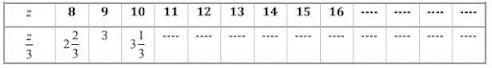(d) Complete the table and by inspection of the table find the solution to the equation m – 7 =3
 m 5 6 7 8 9 10 11 12 13 …. …. m - 7 …. …. …. …. …. …. …. …. …. …. ….

Solution

(a)

 m 1 2 3 4 5 6 7 8 9 10 11 12 13 m + 10 11 12 13 14 15 16 17 18 19 20 21 22 23

∵ At m = 16, m + 10 = 16

∴ m = 6 is the solution.

(b)

 t 3 4 5 6 7 8 9 10 11 12 13 14 15 16 5t 15 20 25 30 35 40 45 50 55 60 65 70 75 80

∵ At t = 7, 5t = 35

∴ t = 7 is the solution.

(c)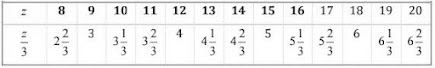∵ At z = 12, z/3 = 4

∴ z = 12 is the solution.

(d)

 m 5 6 7 8 9 10 11 12 13 14 15 m - 7 -2 -1 0 1 2 3 4 5 6 7 8

∵ At m = 10, m – 7 = 3

∴ m = 10 is the solution.